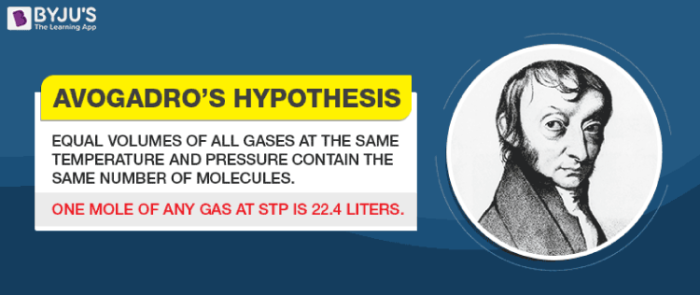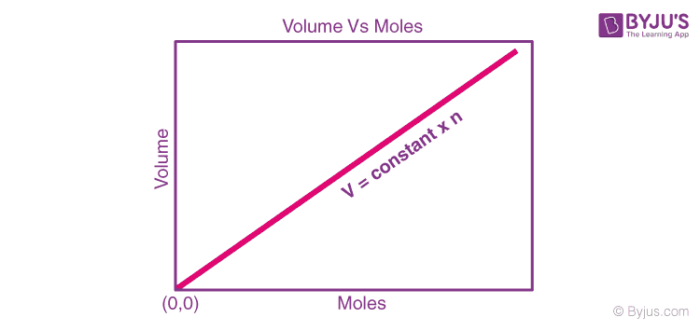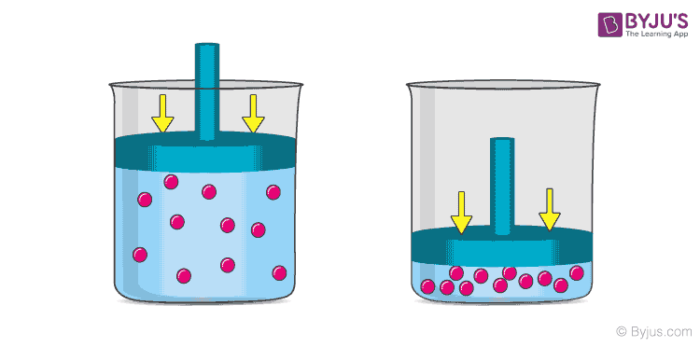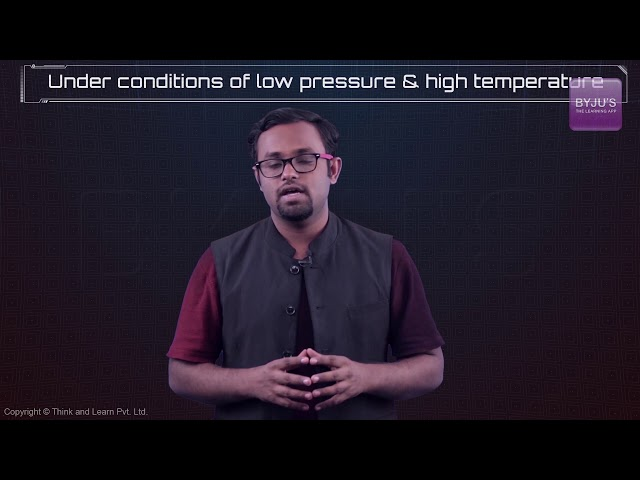Jet Set Go! All about Aeroplanes Jet Set Go! All about Aeroplanes

Avogadro’s law, also known as Avogadro’s principle or Avogadro’s hypothesis, is a gas law which states that the total number of atoms/molecules of a gas (i.e. the amount of gaseous substance) is directly proportional to the volume occupied by the gas at constant temperature and pressure.

Avogadro’s law is closely related to the ideal gas equation since it links temperature, pressure, volume, and amount of substance for a given gas.

## Table of ContentAvogadro’s law is named after the Italian scientist Amedeo Carlo Avogadro, who suggested that two dissimilar ideal gases occupying the same volume at a given (constant) temperature and pressure must contain an equal number of molecules.

## Formula and Graphical Representation

At constant pressure and temperature, Avogadro’s law can be expressed via the following formula:

V ∝ n

V/n = k

Where V is the volume of the gas, n denotes the amount of gaseous substance (often expressed in moles), and k is a constant. When the amount of gaseous substance is increased, the corresponding increase in the volume occupied by the gas can be calculated with the help of the following formula:

V1/n1 = V2/n2 ( = k, as per Avogadro’s law).

The graphical representation of Avogadro’s law (with the amount of substance on the X-axis and volume on the Y-axis) is illustrated below.Here, the straight line (which indicates that the two quantities are directly proportional) passes through the origin, implying that zero moles of gas will occupy zero volume.

## Derivation

Avogadro’s law can be derived from the ideal gas equation, which can be expressed as follows:

PV = nRT

Where,

• ‘P’ is the pressure exerted by the gas on the walls of its container
• ‘V’ is the volume occupied by the gas
• ‘n’ is the amount of gaseous substance (number of moles of gas)
• ‘R’ is the universal gas constant
• ‘T’ is the absolute temperature of the gas

Rearranging the ideal gas equation, the following equation can be obtained.

V/n = (RT)/P

Here, the value of (RT)/P is a constant (since the temperature and pressure kept constant and the product/quotient of two or more constants is always a constant). Therefore:

V/n = k

Thus, the proportionality between the volume occupied by a gas and the number of gaseous molecules is verified.

### Molar Volume of a Gas

As per Avogadro’s law, the ratio of volume and amount of gaseous substance is a constant (at constant pressure and temperature). The value of this constant (k) can be determined with the help of the following equation:

k = (RT)/P

Under standard conditions for temperature and pressure, the value of T corresponds to 273.15 Kelvin and the value of P corresponds to 101.325 kilo Pascals. Therefore, the volume occupied by one mole of a gas at STP is:

Volume occupied by 1 mole of gas = (8.314 J.mol-1.K-1)*(273.15 K)/(101.325 kPa) = 22.4 litres

Therefore, one mole of any gaseous substance occupies 22.4 litres of volume at STP.

The process of respiration is a great example of Avogadro’s law. When humans inhale, the increase in the molar quantity of air in the lungs is accompanied by an increase in the volume of the lungs (expansion of the lungs). An image detailing the change in volume brought on by an increase in the number of gaseous molecules is provided below.Another common example of Avogadro’s law is the deflation of automobile tyres. When the air trapped inside the tyre escapes, the number of moles of air present in the tyre decreases. This results in a decrease in the volume occupied by the gas, causing the tyre to lose its shape and deflate.

## What are the Limitations of Avogadro’s Law?

Despite being perfectly applicable to ideal gases, Avogadro’s law provides only approximate relationships for real gases. The deviation of real gases from ideal behaviour increases at low pressure and high temperature.

It is important to note that gases molecules having relatively low molecular masses (such as helium and hydrogen) obey Avogadro’s law to a greater extent than heavier molecules.

## Solved Exercises on Avogadro’s Law

### Example 1

One mole of helium gas fills up an empty balloon to a volume of 1.5 litres. What would be the volume of the balloon if an additional 2.5 moles of helium gas is added? (Assume that the temperature and the pressure are kept constant)

Given,

The initial amount of helium (n1) = 1 mol

The initial volume of the balloon (V1) = 1.5 L

The final amount of helium (n2) = 1 mol + 2.5 mol = 3.5 mol

As per Avogadro’s law, V1/n1 = V2/n2

Therefore, the final volume of the balloon (V2) = (V1n2)/n1 = (1.5L*3.5mol)/1mol = 5.25 L

The balloon would occupy a volume of 5.25 litres when it contains 3.5 moles of helium gas.

### Example 2

A tyre containing 10 moles of air and occupying a volume of 40L loses half its volume due to a puncture. Considering that the pressure and temperature remain constant, what would be the amount of air in the deflated tyre?

Given,

The initial amount of air (n1) = 10 mol

The initial volume of the tyre (V1) = 40 L

The final volume of the tyre (V2) = 20 L

According to Avogadro’s law, the final amount of air in the tyre (n2) = (V2n1)/V1 = 5 moles.

The deflated tyre would contain 5 moles of air.

## Recommended Videos## Frequently Asked Questions – FAQs

### What does Avogadro’s law state?

Avogadro’s law states that equal volumes of different gases contain an equal number of molecules under the same conditions of temperature and pressure.

### Why is Avogadro’s law important?

The link between the amount of gas (n) and the volume (V) is investigated via Avogadro’s law (v). It’s a direct relationship, which means the volume of a gas is proportional to the number of moles contained in the gas sample. The law is significant because it allows us to save time and money over time.

### What does Charles law state?

The physics theory known as Charles’ law asserts that the volume of a gas equals a constant value multiplied by its Kelvin temperature.

### What is Avogadro’s Law in simple terms?

Avogadro’s law states that equal volumes of different gases contain an equal number of molecules under the same conditions of temperature and pressure.

### Why is Avogadro’s law only for gases?

This is because there is so much space between each molecule that the size of the molecule has no bearing on the volume of the material. This is why the volume of a gas is governed by the pressure applied to it, and why under the same pressure, all gases have the same volume.

### What are the limitations of Avogadro law?

Avogadro law is only applicable to real gases. It is not valid at low temperature and high pressure as a real gas behaves like an ideal gas under that conditions. Moreover, it is also not applicable to heavy molecules.

### What are the applications of Avogadro law?

The Avogadro law is used in determining the molecular formula of a gaseous compound, finding the association between the molecular mass and the vapour density of the gas. It also helps find the association between molecular mass and gas volume at STP.

### Can we apply the ideal gas law to liquids?

No, we can not apply the ideal gas law to liquids. Ideal gas law states that the volume is variable. However, it is not possible in liquids.

### Why was Avogadro’s law rejected?

Avogadro’s law was rejected because, at that time, dalton believed that atoms of the same kind could not combine, which is not true.

1. Aravind

Good

2. ram chander

3. Deborah oluwatoyin

Extraordinarily great

4. Abhinav kakati